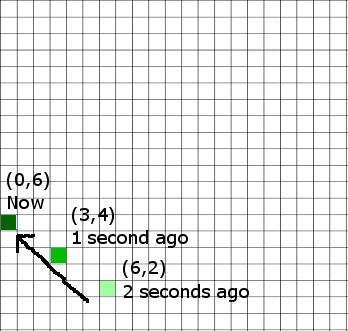## From Pong to Platformers – An Introduction to Game Physics – Part 2

Velocity

Velocity is a fancy word for speed with a particular direction. Going 88 MPH would be speed. Going north at 88MPH would be velocity. But forgetting the direction component for a moment, speed is measured in distanced traveled over time. For example, when we say 88 Miles per Hour, we’re saying the object is moving a distance of 88 miles over a period of an hour – i.e. the object is changing its position by that much in an hour.

Remember, our video game object has position too – it has its coordinates. If we introduce velocity into our game, we now give our object the ability to move by the laws of physics. If we redraw our object once a second, and the object is going 5 pixels right per second, we would be adding 5 to our X coordinate (the value stored in our X variable) every second, then redrawing the object. If we want it to move faster, we increase the speed, 6 pixels per second, 7 pixels per second.

Don’t Forget the Vector!

Vector is another mathematical term for a quantity with a direction involved. Remember, velocity is speed with a direction, so it is a vector. There is a helpful property of vectors that allows us to split them up by dimensions – that is, if something is moving in two dimensions at once (north and west, up and north, south and east and down), we can handle each direction separately. If something is moving north and west, we can talk about how much it’s moving in the north direction and how much it’s moving in the east directly independently. In our video game case, we can say “okay, the pong ball is traveling -3 pixels per second in the X direction, and 2 pixels per second in the Y direction). That means every second we’re going to subtract 3 from our X variable, and add 2 to our Y variable, then redraw the ball. The movement of the ball would appear to be going diagonally up and left, as seen here:We handled each dimension separately, but the end result was the diagonal movement.

Now we can store our X velocity and Y velocity into variables too (for example, velX and velY). So, every time we redraw the screen, we are going to add velX to X (x = x + velX), and velY to Y (y = y + velY). So if we have a very large velX, the ball will be moved across the screen very fast from left to right. If we have a very large negative velY, the ball will be moved down the screen very fast. And we don’t need to press the key each time, our velocity will do the work for us through simple addition (or subtraction if we are adding a negative number).

If we wanted to design a fun experiment, we could assign our arrow keys to change velocity. Pressing left would subtract one from velocity in the X direction, pressing up would add one to velocity in the y direction, etc. This would have the effect of making the ball move in a direction more quickly the more you press the arrow in the same direction, or slow down and start moving backwards if you press the arrow in the opposite direction. Not pressing the arrows would simply keep the ball doing whatever it was doing – e.g. traveling in whatever direction it was before.

Onto Part 3

### One Response to From Pong to Platformers – An Introduction to Game Physics – Part 2

1.ravi teja says:

its nice, and clear. but if u give the code how to implement this its better to understand clearly.if i want the ball is moving some distance in some speed and some distance in some other speed then what to do. if ball touches the ground i want turn the ball into some direction with angle like in the cricket, when the ball reaches the ground it reflects. in these situations what to do, the ball positions are different from previous ball positions. the speed is based on the pixels how much we give am i right. then i increase the X then what about Y how to increase or decrease that.